# NCERT Based Test: Kossel-Lewis Approach to Chemical Bonding

## 10 Questions MCQ Test NCERTs for NEET | NCERT Based Test: Kossel-Lewis Approach to Chemical Bonding

Description
Attempt NCERT Based Test: Kossel-Lewis Approach to Chemical Bonding | 10 questions in 10 minutes | Mock test for NEET preparation | Free important questions MCQ to study NCERTs for NEET for NEET Exam | Download free PDF with solutions
QUESTION: 1

### The electronic configuration of four atoms are given in brackets: L(1s22s22p1);  M(1s22s22p5); Q(1s22s22p63s1); R(1s22s22p2); The element that would most readily form a diatomic molecule is

Solution:

By sharing of 1electron each, both the atoms of M will get a completed octet.

QUESTION: 2

### Two elements P and Q combine to form a compound. If P has 2 and Q has 6 electrons in their outermost shell, what will be formula of the compound formed?

Solution:

Valence electrons in P is 2; Valence electrons in Q is 6.

P - 2e- → P2+

Q + 2e- → Q2-

Since both show a valency of 2, the formula for the compound will be PQ.

QUESTION: 3

### How many number of electrons are involved in the formation of a nitrogen molecule?

Solution: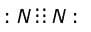Number of electrons involved in bonding is 6.

QUESTION: 4

In which of the following molecules octet rule is not followed?

Solution: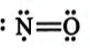For NO, the octet rule is not followed due to the presence of odd electrons on N.

QUESTION: 5

A pair of electrons present between two identicalnon-metals.

Solution:

An electron pair is equally shared between two identical atoms like F — F, H — H.
It is pulled towards a more electronegative atom in case of dissimilar atoms.

QUESTION: 6

During a coordinate bond formation,

Solution:

In a coordinate or dative bond, electrons required for sharing are contributed by one atom. The atom which contributes the electrons for sharing is called donor atom and the atom which shares the electrons is called acceptor atom.

QUESTION: 7

Which of the following molecules contains covalent and coordinate bonds?

Solution: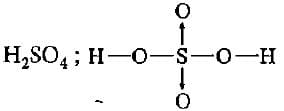QUESTION: 8

Which of the following shows the Lewis dot formula for CO2?

Solution:

Step I: Skeleton OCO

Step II: A = 1 x 4 for C + 2 x 6 for O = 4 + 12 = 16 electrons

Step III : Total no. of electrons needed to achieve noble gas configuration (N)
N= 1 x 8 + 2 x 8 = 24

Step IV : Shared electrons, S = N- A = 24-16 = 8 electrons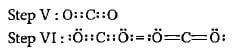QUESTION: 9

How many and what types of bonds are present in NH4+?

Solution: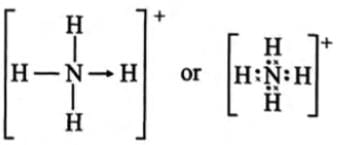QUESTION: 10

What is the formal charge on carbon atom in the following two structures?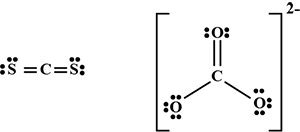Solution:

Formal charge on C atom = Valence electrons
-Lone pair of electrons −1/2× Bonding electrons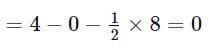formal charge on C atom =0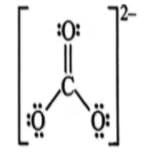Use Code STAYHOME200 and get INR 200 additional OFF Use Coupon Code